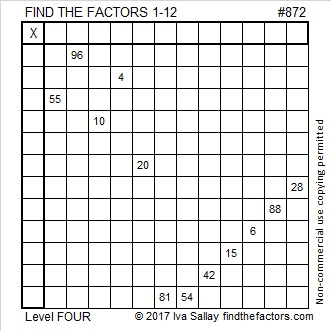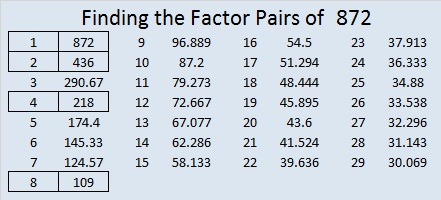# 872 and Level 4

26² + 14² = 872 so 872 is the hypotenuse of a Pythagorean triple:

480-728-872 which can be calculated from 26² – 14², 2(26)(14), 26² + 14².

872 is the sum of consecutive prime numbers 433 and 439.

872 is also the sum of the 21 prime numbers from 3 to 79.

872! + 1 is a number much too big for any calculator I own, but OEIS.org informs us that it is a prime number.Print the puzzles or type the solution on this excel file: 12 factors 864-874

• 872 is a composite number.
• Prime factorization: 872 = 2 × 2 × 2 × 109, which can be written 872 = 2³ × 109
• The exponents in the prime factorization are 3 and 1. Adding one to each and multiplying we get (3 + 1)(1 + 1) = 4 × 2 = 8. Therefore 872 has exactly 8 factors.
• Factors of 872: 1, 2, 4, 8, 109, 218, 436, 872
• Factor pairs: 872 = 1 × 872, 2 × 436, 4 × 218, or 8 × 109
• Taking the factor pair with the largest square number factor, we get √872 = (√4)(√218) = 2√218 ≈ 29.529646